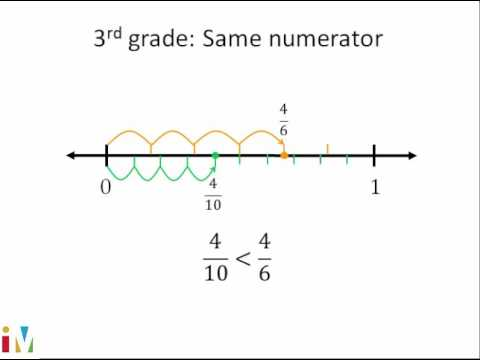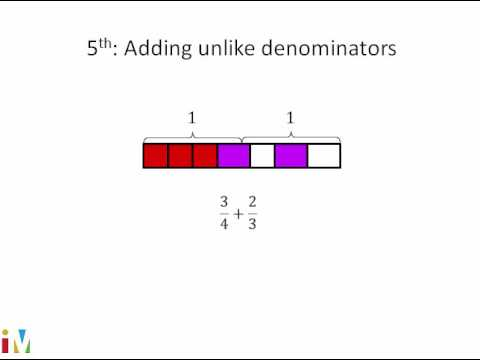Video

# Subtracting mixed numbers with like denominators (Full video)

Description: Sal solves a word problem involving mixed number subtraction by using a visual model. So here are three different puddles and she measures the depth in inches. So they say how much deeper was the puddle under the swing, so that's this one right over here it's one and 1/4 inches deep it's under the swing. And we see here the puddle on the sidewalk is 2/4 inches deep, so what we could do is subtract the 2/4 from the one and 1/4.

### Other videos you might be interested in### Comparing Fractions

#### Illustrative Mathematics